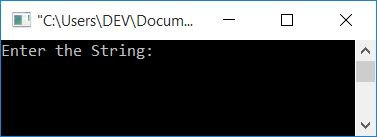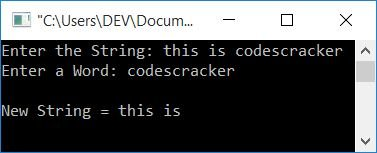# C Program to Delete a Word from a String

In this article, you will learn and get code for deleting a word from a string. Here, the program will ask the user to enter any string and then a word to delete the given word from the given string. The program is created with and without using a two-dimensional array.

## In C, delete a word from a string

To delete a particular word from the given string in C programming, you have to ask the user to enter the string and then ask for the word that has to be deleted. Then check for the presence of the given word in the string. If found, then delete it as shown in the program given below:

```#include<stdio.h>
#include<conio.h>
#include<string.h>
int main()
{
char str, word;
int i, j, ls, lw, temp, chk=0;
printf("Enter the String: ");
gets(str);
printf("Enter a Word: ");
gets(word);
ls = strlen(str);
lw = strlen(word);
for(i=0; i<ls; i++)
{
temp = i;
for(j=0; j<lw; j++)
{
if(str[i]==word[j])
i++;
}
chk = i-temp;
if(chk==lw)
{
i = temp;
for(j=i; j<(ls-lw); j++)
str[j] = str[j+lw];
ls = ls-lw;
str[j]='\0';
}
}
printf("\nNew String = %s", str);
getch();
return 0;
}```

This program was built and runs under the Code::Blocks IDE. Here is its sample run:Now enter any string, say "this is codescracker," followed by a word, say "codescracker." Press the `ENTER` key to see the following output:In the above program, the following code fragment:

```for(j=0; j<lw; j++)
{
if(str[i]==word[j])
i++;
}```

is used to check for the presence of a word (that has to be deleted) with character-by-character matching. But before the above code fragment, the value of i  was initialized with temp. So that after the code fragment, we can check whether the "if" statement gets evaluated for the number of times that equals the length of the word or not. If it is, then the word is found. As a result, the following code fragment:

```for(j=i; j<(ls-lw); j++)
str[j] = str[j+lw];```

is used to move all the characters (after the last character's index of the word) to the place from the word where the first character's index is in the string. That is, if the word is found in the given string at index number 5. And the length of the word is 3, therefore we have to move the character at the 8th index to the 5th, the character at the 9th index to the 6th index, and so on up to the last character of the string.

Never forget to initialize the new length of the string to the ls variable. Every time the word is deleted (if it appears more than once), simply divide the length of the string by the length of the word. Now add a null-terminated character (\0) to the last index of the new string.

This program has a limitation. For example, if the string is "this is codescracker," and the user wants to delete "is," Then "is" from "this" also gets deleted. Therefore, the string becomes "th codescracker" after deleting "is." To overcome this problem, we have another program, as given below:

### Complete Version of the Deleting Word from a String Program

Here is the complete version of the previous program. This program also deletes duplicate spaces from the final string.

```#include<stdio.h>
#include<conio.h>
#include<string.h>
int main()
{
char str, word;
int i, j, ls, lw, temp, chk=0, doIncrement, isSpace;
printf("Enter the String: ");
gets(str);
printf("Enter a Word: ");
gets(word);
ls = strlen(str);
lw = strlen(word);
for(i=0; i<ls; i++)
{
temp = i;
doIncrement = 0;
for(j=0; j<lw; j++)
{
if(str[i]==word[j])
{
if(temp>0 && (temp+lw)<ls)
{
if(str[temp-1]== ' ' && str[temp+lw]==' ')
doIncrement=1;
}
else if(temp==0 && (temp+lw)<ls)
{
if(str[temp+lw]==' ')
doIncrement=1;
}
else if(temp>0 && (temp+lw)==ls)
{
if(str[temp-1]== ' ')
doIncrement=1;
}
if(doIncrement==1)
i++;
else
break;
}
}
chk = i-temp;
if(chk==lw)
{
i = temp;
for(j=i; j<(ls-lw); j++)
str[j] = str[j+lw];
ls = ls-lw;
i = temp;
str[j]='\0';
}
}
ls = strlen(str);
i=0;
while(str[i]!='\0')
{
isSpace = 0;
if(str[i]==' ' && str[i+1]==' ')
{
for(j=i; j<(ls-1); j++)
{
str[j] = str[j+1];
isSpace = 1;
}
}
if(i==0 && str[i]==' ')
{
for(j=i; j<(ls-1); j++)
{
str[j] = str[j+1];
isSpace = 1;
}
}
if(isSpace==0)
i++;
else
{
str[j]='\0';
ls--;
}
}
printf("\nNew String = %s", str);
getch();
return 0;
}```

Now let's provide the input string as "is this is is is isth codescracker" and then "is" as the word to delete. Here is its sample run:Here the words "this" and "isth" are included to check whether the word "is" (to be deleted) gets matched from any of the sides or not. As you can see from both sides, "is" does match with both the words "this" and "isth" and also deletes all the extra spaces.

### Using a Two-Dimensional Array, Remove a Word from a String

Now let's create the same-purpose program using a two-dimensional array. All of the words in the given string are placed one by one in a two-dimensional array called stringIn2DArray[]. And then I checked for the word "to be deleted." If found, just perform the task of deleting it as shown here:

```#include<stdio.h>
#include<conio.h>
#include<string.h>
int main()
{
char str, word, stringIn2DArray;
int i, j=0, k=0;
printf("Enter the String: ");
gets(str);
printf("Enter the Word (to be Delete): ");
gets(word);
for(i=0; str[i]!='\0'; i++)
{
if(str[i]==' ')
{
stringIn2DArray[k][j]='\0';
k++;
j=0;
}
else
{
stringIn2DArray[k][j]=str[i];
j++;
}
}
stringIn2DArray[k][j] = '\0';
j=0;
for(i=0; i<(k+1); i++)
{
if(!strcmp(stringIn2DArray[i], word))
stringIn2DArray[i][j]='\0';
}
printf("\nThe New String is: ");
j=0;
for(i=0; i<(k+1); i++)
{
if(stringIn2DArray[i][j] == '\0')
continue;
else
printf("%s ", stringIn2DArray[i]);
}
getch();
return 0;
}```

This program produces the same output as the previous one.

#### The same program in different languages

C Quiz

« Previous Program Next Program »